Get inspired by the success stories of our students in IIT JAM MS, ISI  MStat, CMI MSc DS.  Learn More

# Cumulative Distributive Function | ISI M.Stat 2019 PSB Problem B6This problem based on Cumulative Distributive Function gives a detailed solution to ISI M.Stat 2019 PSB Problem 6, with a tinge of simulation and code.

## Problem

Suppose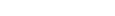is a random sample from Uniform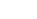for some unknown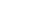. Let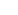be the minimum of.
(a) Suppose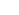is the cumulative distribution function (c.d.f.) of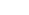.
Show that for any real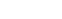converges to, where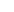is the c.d.f. of an exponential distribution with mean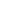.
(b) Find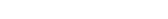for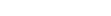where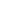denotes the largest integer less than or equal to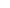.

## Solution

#### Distribution of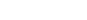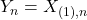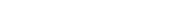from the results of the order statistics

Now, let's compute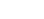.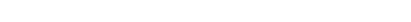.

Observe that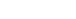. We will need it in the second part.

So,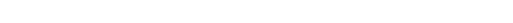, where~ exp(mean).

Let's add a computing dimension to it, we will verify the result using simulation.

Let's take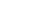.

• Generateis a random sample from Uniform. n = 100000.
• Select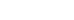. Calculate.
• Repeat this process k = 1000 times.
• Plot the histogram.
• Plot and match with exp(mean).
v = NULL
for (i in 1:100000) {
r  = runif(100, 0, 2)
m = 100*min(r)
v = c(v,m)
}
hist(v, freq = FALSE)
x = seq(0, 10, 0.0001)
curve(dexp(x, 0.5), from = 0, col = "red", add = TRUE)
require(vcd)
require(MASS)
#Fitting the data with exponential distribution
fit <- fitdistr(v, "exponential")
fit
# rate = 0.504180128 ( which is close to 0.5 = the actual rate)

The following is the diagram.

We need to compute thisSo, observe that for any fixed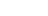,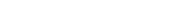.

Hence,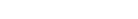. Hence, for k > 0,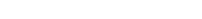.

Let's compute separately for k = 0.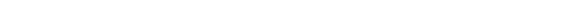.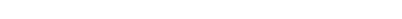.

Thus, this problem is just an application of the first part.

This problem based on Cumulative Distributive Function gives a detailed solution to ISI M.Stat 2019 PSB Problem 6, with a tinge of simulation and code.

## Problem

Supposeis a random sample from Uniformfor some unknown. Letbe the minimum of.
(a) Supposeis the cumulative distribution function (c.d.f.) of.
Show that for any realconverges to, whereis the c.d.f. of an exponential distribution with mean.
(b) Findforwheredenotes the largest integer less than or equal to.

## Solution

#### Distribution offrom the results of the order statistics

Now, let's compute..

Observe that. We will need it in the second part.

So,, where~ exp(mean).

Let's add a computing dimension to it, we will verify the result using simulation.

Let's take.

• Generateis a random sample from Uniform. n = 100000.
• Select. Calculate.
• Repeat this process k = 1000 times.
• Plot the histogram.
• Plot and match with exp(mean).
v = NULL
for (i in 1:100000) {
r  = runif(100, 0, 2)
m = 100*min(r)
v = c(v,m)
}
hist(v, freq = FALSE)
x = seq(0, 10, 0.0001)
curve(dexp(x, 0.5), from = 0, col = "red", add = TRUE)
require(vcd)
require(MASS)
#Fitting the data with exponential distribution
fit <- fitdistr(v, "exponential")
fit
# rate = 0.504180128 ( which is close to 0.5 = the actual rate)

The following is the diagram.

We need to compute thisSo, observe that for any fixed,.

Hence,. Hence, for k > 0,.

Let's compute separately for k = 0...

Thus, this problem is just an application of the first part.

This site uses Akismet to reduce spam. Learn how your comment data is processed.

### Knowledge Partner